# Source https en wikipedia orgwikiInsertionsortmediaFile Insertionsort gif Source

• Slides: 24Source: https: //en. wikipedia. org/wiki/Insertion_sort#/media/File: Insertion_sort. gifSource: http: //math. hws. edu/eck/cs 124/javanotes 3/c 8/fig 4. gifInsertion Sort for (int i = 1; i < n; i++) {// insert a[i] into a[0: i-1] int t = a[i]; int j; for (j = i - 1; j >= 0 && t < a[j]; j--) a[j + 1] = a[j]; a[j + 1] = t; }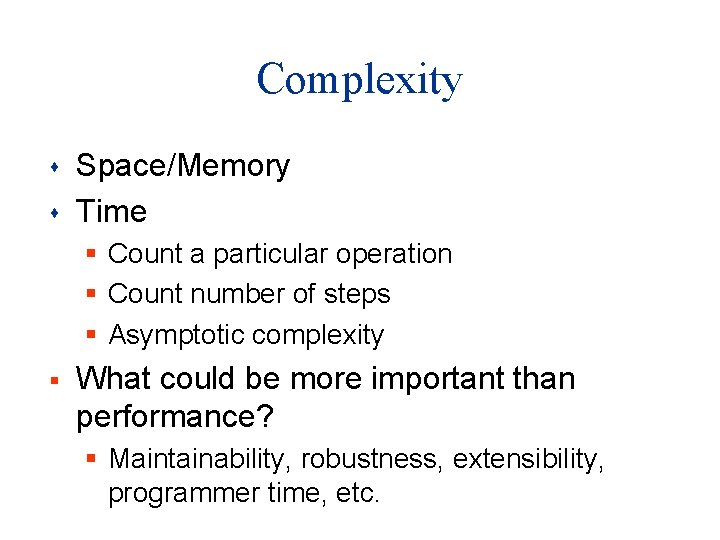Complexity s s Space/Memory Time § Count a particular operation § Count number of steps § Asymptotic complexity § What could be more important than performance? § Maintainability, robustness, extensibility, programmer time, etc.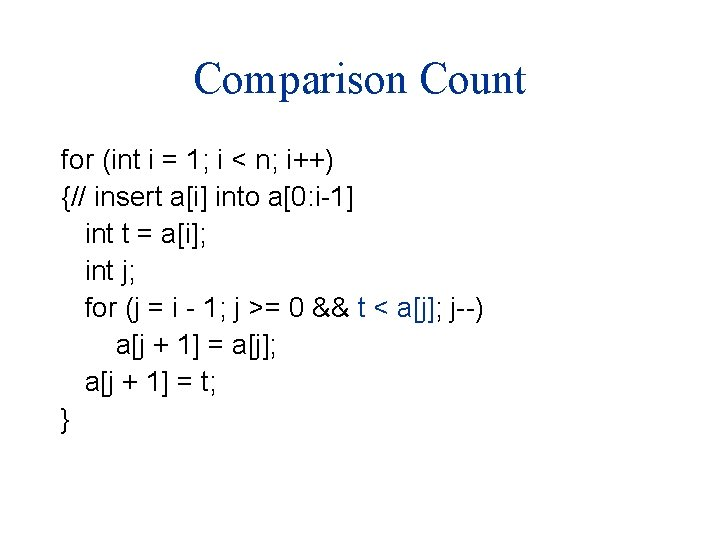Comparison Count for (int i = 1; i < n; i++) {// insert a[i] into a[0: i-1] int t = a[i]; int j; for (j = i - 1; j >= 0 && t < a[j]; j--) a[j + 1] = a[j]; a[j + 1] = t; }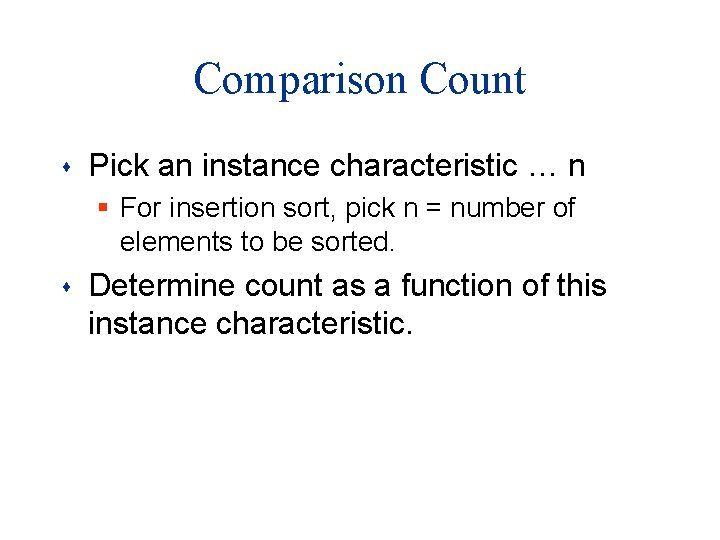Comparison Count s Pick an instance characteristic … n § For insertion sort, pick n = number of elements to be sorted. s Determine count as a function of this instance characteristic.Comparison Count for (j = i - 1; j >= 0 && t < a[j]; j--) a[j + 1] = a[j]; How many comparisons are made?Comparison Count for (j = i - 1; j >= 0 && t < a[j]; j--) a[j + 1] = a[j]; number of compares depends on a[]s and t as well as on i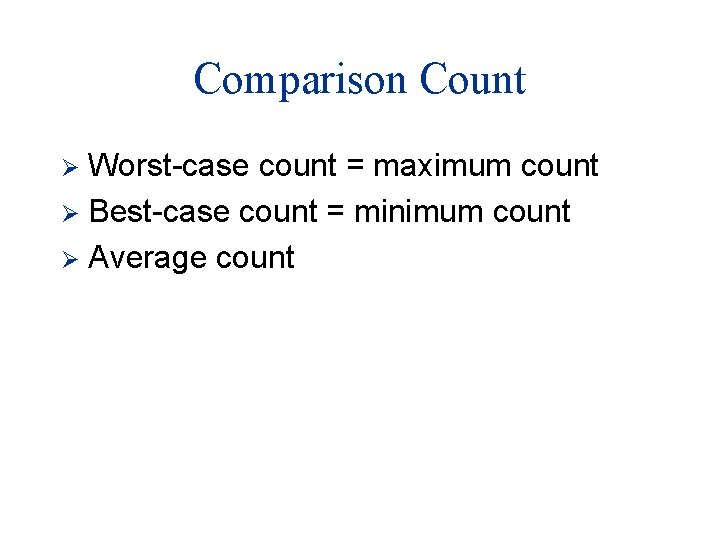Comparison Count Worst-case count = maximum count Ø Best-case count = minimum count Ø Average count Ø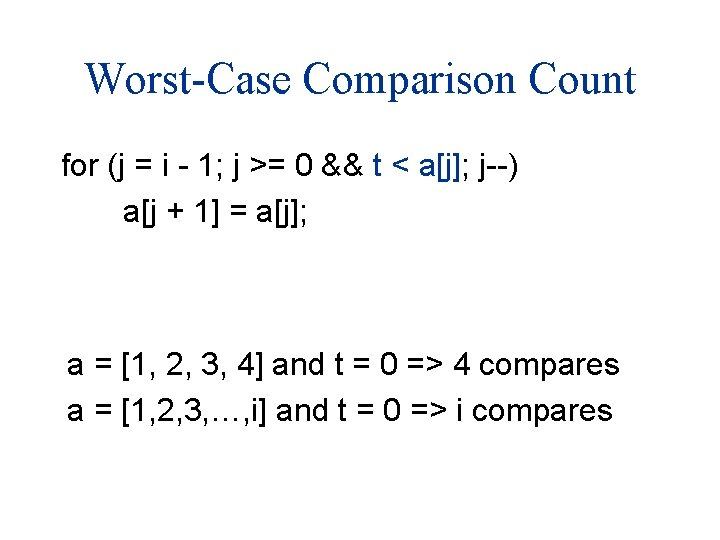Worst-Case Comparison Count for (j = i - 1; j >= 0 && t < a[j]; j--) a[j + 1] = a[j]; a = [1, 2, 3, 4] and t = 0 => 4 compares a = [1, 2, 3, …, i] and t = 0 => i comparesWorst-Case Comparison Count for (int i = 1; i < n; i++) for (j = i - 1; j >= 0 && t < a[j]; j--) a[j + 1] = a[j]; total compares = 1 + 2 + 3 + … + (n-1) = (n-1)n/2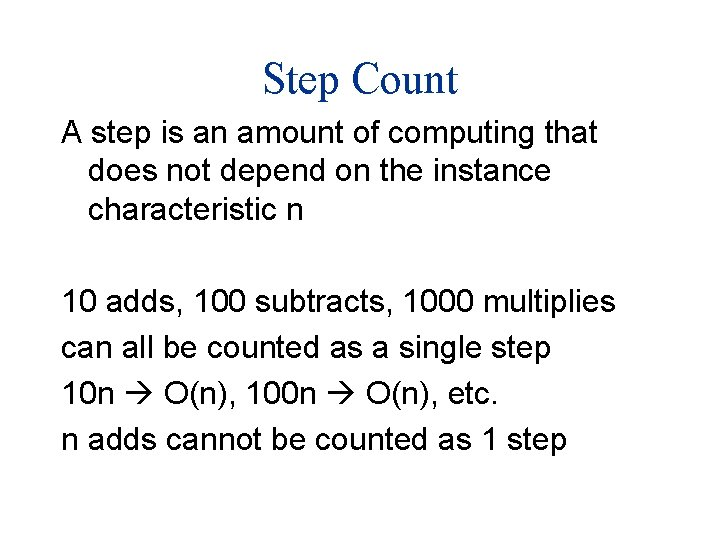Step Count A step is an amount of computing that does not depend on the instance characteristic n 10 adds, 100 subtracts, 1000 multiplies can all be counted as a single step 10 n O(n), 100 n O(n), etc. n adds cannot be counted as 1 step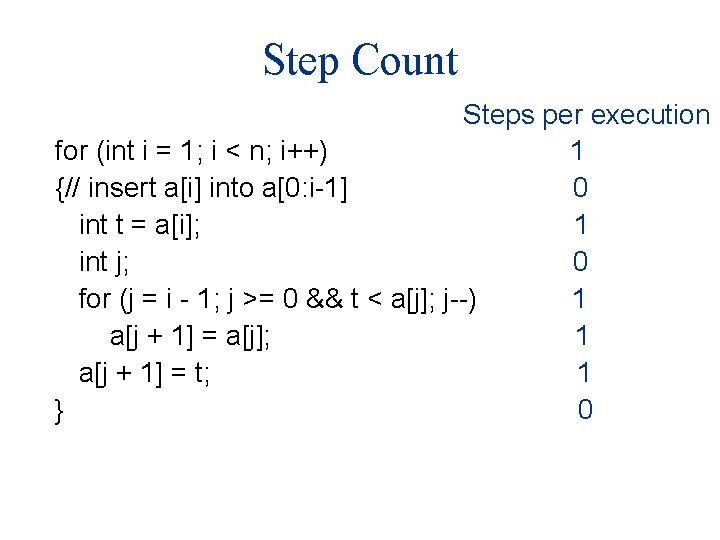Step Count Steps per execution for (int i = 1; i < n; i++) 1 {// insert a[i] into a[0: i-1] 0 int t = a[i]; 1 int j; 0 for (j = i - 1; j >= 0 && t < a[j]; j--) 1 a[j + 1] = a[j]; 1 a[j + 1] = t; 1 } 0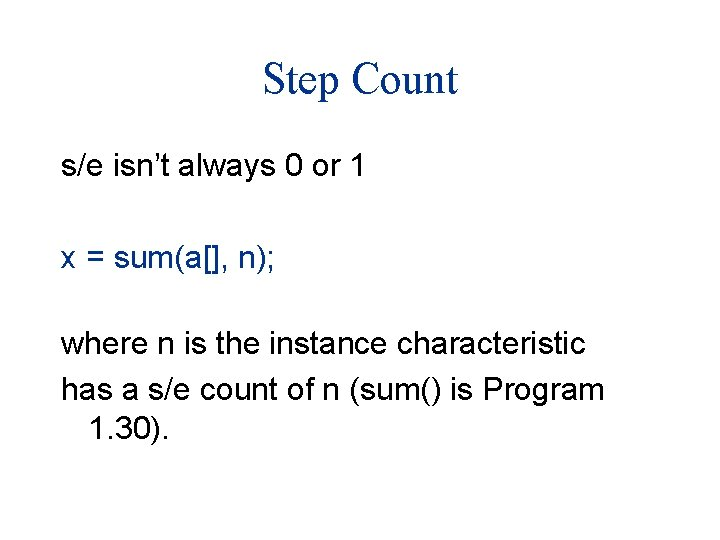Step Count s/e isn’t always 0 or 1 x = sum(a[], n); where n is the instance characteristic has a s/e count of n (sum() is Program 1. 30).Step Count for (int i = 1; i < n; i++) {// insert a[i] into a[0: i-1] int t = a[i]; int j; for (j = i - 1; j >= 0 && t < a[j]; j--) a[j + 1] = a[j]; a[j + 1] = t; } s/e steps 1 0 i+ 1 1 1 i 1 0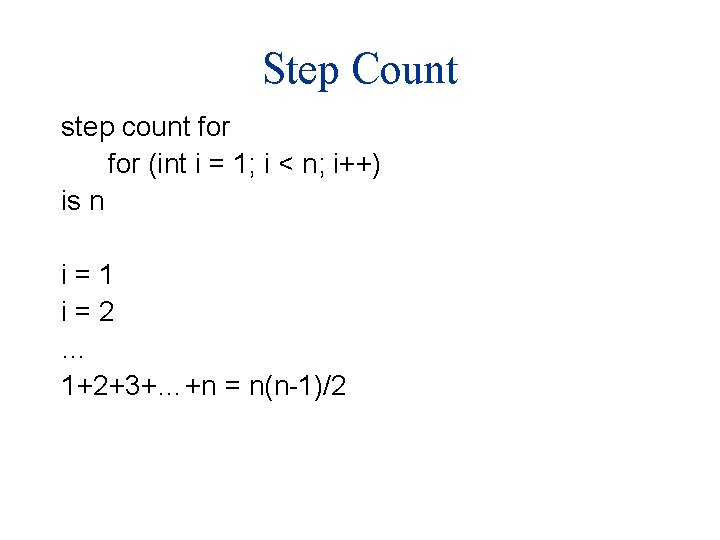Step Count step count for (int i = 1; i < n; i++) is n i=1 i=2 … 1+2+3+…+n = n(n-1)/2Space Complexity s s O(n) total O(1) additional space Source: https: //en. wikipedia. org/wiki/Insertion_sort#/media/File: Insertion-sort-example-300 px. gif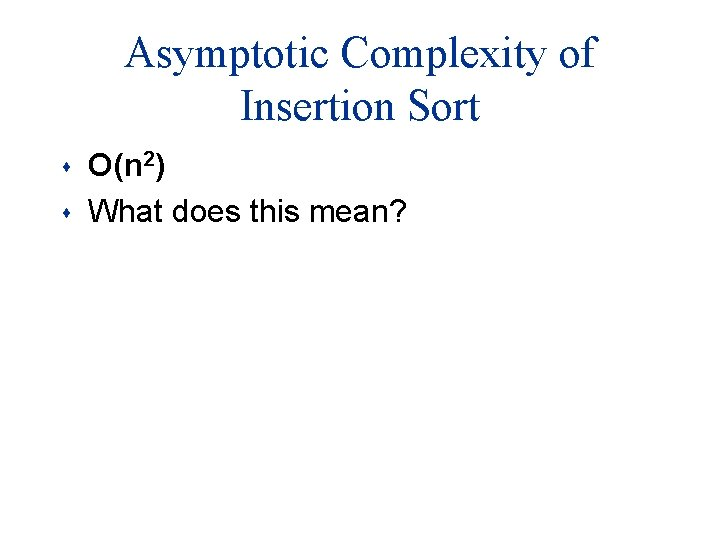Asymptotic Complexity of Insertion Sort s s O(n 2) What does this mean?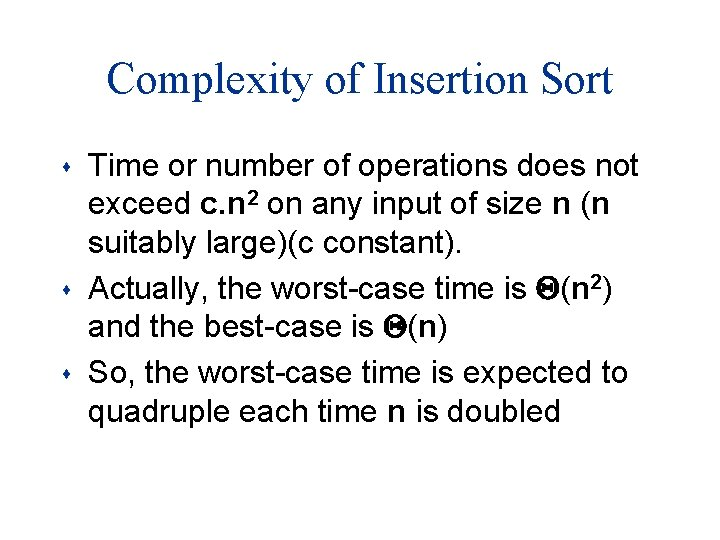Complexity of Insertion Sort s s s Time or number of operations does not exceed c. n 2 on any input of size n (n suitably large)(c constant). Actually, the worst-case time is Q(n 2) and the best-case is Q(n) So, the worst-case time is expected to quadruple each time n is doubledComplexity of Insertion Sort s s Is O(n 2) too much time? Is the algorithm practical?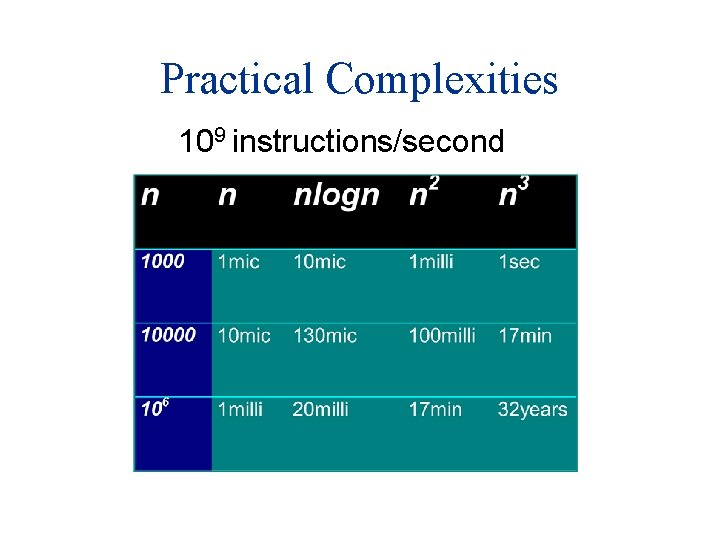Practical Complexities 109 instructions/secondImpractical Complexities 109 instructions/second Age of the universe: 13. 8 * 10^9 years!*Faster Computer Vs Better Algorithmic improvement more useful than hardware improvement. E. g. 2 n to n 3To-do s Read: – https: //en. wikipedia. org/wiki/Big_O_notation s Do: – Create Git. Hub (or similar) account – Insert 0 into 1 2 3 4 5 – Count Steps – Insert 10 into 1 2 3 4 5 – Count Steps s Implement (ungraded): – Insertion Sort (C++) – Sort a sorted sequence/reversely sorted sequence (n=1000, 1000000), what’s the difference in runtime?